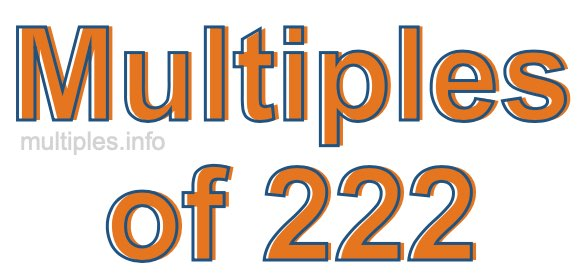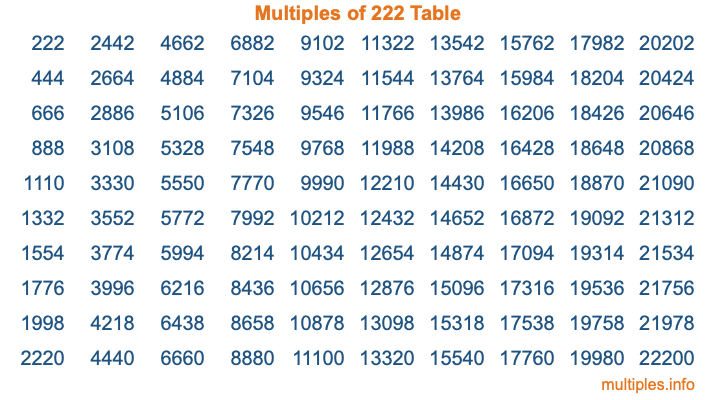Multiples of 222Welcome to the Multiples of 222 page. Here we will first teach you everything you will ever need to know about the multiples of 222, and then give you a study guide summary of everything we taught you to make sure you remember it all. Use this page to look up facts and learn information about the multiples of 222. This page will make you a multiples of two hundred twenty-two expert!

Definition of Multiples of 222
Multiples of 222 are all the numbers that when divided by 222 equal an integer. Each of the multiples of 222 are called a multiple. A multiple of 222 is created by multiplying 222 by an integer.

Therefore, to create a list of multiples of 222, you start with 1 multiplied by 222, then 2 multiplied by 222, then 3 multiplied by 222, and so on for as long as you want. Thus, the list of the first five multiples of 222 is 222, 444, 666, 888, and 1110. To see a larger list of multiples of 222, see the printable image of Multiples of 222 further down on this page. We also have a category where you can choose any nth multiple of 222.

Multiples of 222 Checker
The Multiples of 222 Checker below checks to see if any number of your choice is a multiple of 222. In other words, it checks to see if there is any number (integer) that when multiplied by 222 will equal your number. To do that, we divide your number by 222. If the the quotient is an integer, then your number is a multiple of 222.

Is  a multiple of 222?

Least Common Multiple of 222 and ...
A Least Common Multiple (LCM) is the lowest multiple that two or more numbers have in common. This is also called the smallest common multiple or lowest common multiple and is useful to know when you are adding our subtracting fractions. Enter one or more numbers below (222 is already entered) to find the LCM.

Check out our LCM Calculator if you need more details about the Least Common Multiple or if you need the LCM for different numbers for adding and subtraction fractions.

nth Multiple of 222
As we stated above, 222 is the first multiple of 222, 444 is the second multiple of 222, 666 is the third multiple of 222, and so on. Enter a number below to find the nth multiple of 222.

th multiple of 222

Multiples of 222 vs Factors of 222
222 is a multiple of 222 and a factor of 222, but that is where the similarities end. All postive multiples of 222 are 222 or greater than 222. All positive factors of 222 are 222 or less than 222.

Below is the beginning list of multiples of 222 and the factors of 222 so you can compare:

Multiples of 222: 222, 444, 666, 888, 1110, etc.

Factors of 222: 1, 2, 3, 6, 37, 74, 111, 222

As you can see, the multiples of 222 are all the numbers that you can divide by 222 to get a whole number. The factors of 222, on the other hand, are all the whole numbers that you can multiply by another whole number to get 222.

It's also interesting to note that if a number (x) is a factor of 222, then 222 will also be a multiple of that number (x).

Multiples of 222 vs Divisors of 222
The divisors of 222 are all the integers that 222 can be divided by evenly. Below is a list of the divisors of 222.

Divisors of 222: 1, 2, 3, 6, 37, 74, 111, 222

The interesting thing to note here is that if you take any multiple of 222 and divide it by a divisor of 222, you will see that the quotient is an integer.

Multiples of 222 Table
Below is an image of the first 100 multiples of 222 in a table. The table is in chronological order, column by column. The first column has the first ten multiples of 222, the second column has the next ten multiples of 222, and so on.The Multiples of 222 Table is also referred to as the 222 Times Table or Times Table of 222. You are welcome to print out our table for your studies.

Negative Multiples of 222
Although not often discussed or needed in math, it is worth mentioning that you can make a list of negative multiples of 222 by multiplying 222 by -1, then by -2, then by -3, and so on, to get the following list of negative multiples of 222:

-222, -444, -666, -888, -1110, etc.

Multiples of 222 Summary
Below is a summary of important Multiples of 222 facts that we have discussed on this page. To retain the knowledge on this page, we recommend that you read through the summary and explain to yourself or a study partner why they hold true.

There are an infinite number of multiples of 222.

A multiple of 222 divided by 222 will equal a whole number.

222 divided by a factor of 222 equals a divisor of 222.

The nth multiple of 222 is n times 222.

The largest factor of 222 is equal to the first positive multiple of 222.

222 is a multiple of every factor of 222.

222 is a multiple of 222.

A multiple of 222 divided by a divisor of 222 equals an integer.

222 divided by a divisor of 222 equals a factor of 222.

Any integer times 222 will equal a multiple of 222.

Multiples of a Number
Here you can get the multiples of another number, all with the same attention to detail as we did for multiples of 222 on this page.

Multiples of
Multiples of 223
Did you find our page about multiples of two hundred twenty-two educational? Do you want more knowledge? Check out the multiples of the next number on our list!